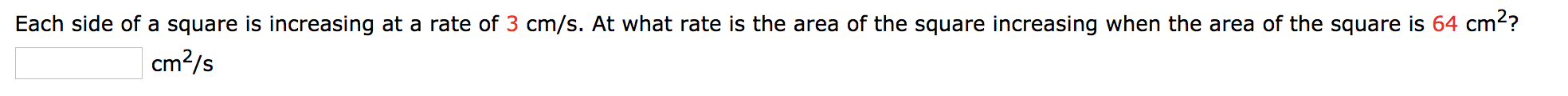# Each side of a square is increasing at a rate of 3 cm/s. At what rate is the area of the square increasing when the area of the square is 64 cm2?cm2/s

Questionhelp_outlineImage TranscriptioncloseEach side of a square is increasing at a rate of 3 cm/s. At what rate is the area of the square increasing when the area of the square is 64 cm2? cm2/s fullscreen
check_circleExpert Solution
Step 1

Given:

Each side of a square is increasing at a rate of 3 cm/s. At what rate is the area of the square increasing when the area of the square is 64 cm2?

Step 2

The given information can be seen from the below figure.

Step 3

So, from the figure and given...

### Want to see the full answer?

See Solution

#### Want to see this answer and more?

Solutions are written by subject experts who are available 24/7. Questions are typically answered within 1 hour*

See Solution
*Response times may vary by subject and question
Tagged in

### Derivative